# Aleph

(diff) ← Older revision | Latest revision (diff) | Newer revision → (diff)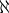The first letter of the Hebrew alphabet. As symbols alephs were introduced by G. Cantor to denote the cardinal numbers (the cardinality cf. Cardinal number) of infinite well-ordered sets. Each cardinal number is some aleph (a consequence of the axiom of choice). However, many theorems about alephs are demonstrated without recourse to the axiom of choice. For each ordinal numberbyone denotes the cardinality of the set of all ordinal numbers smaller than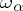. In particular,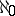is the cardinality of the set of all natural numbers,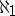is the cardinality of the set of all countable ordinal numbers, etc. If, then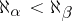. The cardinal numberis the smallest cardinal number which follows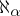. The generalized continuum hypothesis states thatfor any ordinal number. If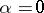, the equation assumes the form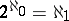, and forms the content of the continuum hypothesis. The set of all alephs smaller thanis totally ordered according to magnitude, and its order type is. The definitions of the sum, the product and a power of alephs are obvious. One hasThe following formulas are most-frequently encountered. The recursive Hausdorff formula: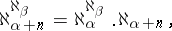a particular case of which, for, is the Bernshtein formula: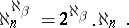The recursive formula of Tarski: If an ordinal numberis a limit ordinal, and if, thenHeredenotes the confinal character of the ordinal number. As in the case of cardinal numbers, one distinguishes between singular alephs, regular alephs, limit alephs, weakly inaccessible alephs, strongly inaccessible alephs, etc. For example,is singular ifis a limit ordinal and if. There is no largest aleph among all alephs. It was shown by Cantor that the set of all alephs is meaningless, i.e. that there is no such set. See also Totally well-ordered set; Continuum hypothesis; Set theory; Ordinal number; Cardinal number.

How to Cite This Entry:
Aleph. Encyclopedia of Mathematics. URL: http://encyclopediaofmath.org/index.php?title=Aleph&oldid=18544
This article was adapted from an original article by B.A. Efimov (originator), which appeared in Encyclopedia of Mathematics - ISBN 1402006098. See original article